# Pine

How much of a mixed forest rangers want to cut down, if their head said:

"We only cut down pine trees, which in our mixed forest 98%. After cut pine trees constitute 94% of all trees left."

p =  66.67 %

### Step-by-step explanation:

$p=100\cdot \left(1-\frac{100-98}{100-94}\right)=66.67\mathrm{%}$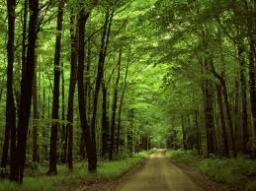Did you find an error or inaccuracy? Feel free to write us. Thank you!Tips to related online calculators
Do you have a linear equation or system of equations and looking for its solution? Or do you have a quadratic equation?

## Related math problems and questions:

• Geography testsOn three 150-point geography tests, you earned grades of 88%, 94%, and 90%. The final test is worth 250 points. What percent do you need on the final to earn 93% of the total points on all tests?
• Water in vegetables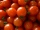Tomatoes in the store contain 99% water. After being transported to the shop, they were slightly dried and contained only 98% of water. How many kgs of tomatoes are in the store if there were 300 kg in stock?`
• Class enrollmentA grade 6 class went on a field trip only 98% or 49 pupils joined the activity. What was the class enrollment?
• Mushrooms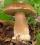Fresh mushrooms contain 94% water, dried 14%. How many kg of fresh mushrooms is needed to collect to get 10 kg dried?
• Pine's forest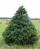There were so many pines in the forest that they were sequentially numbered 1, 2, 3,..., would use three times more digits than the pine trees alone. How many pine trees were there in the forest?
• Cut trees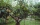There are 1,200 trees in the grove, of which 55 percent are deciduous, the remaining coniferous. Workers cut down 35 percent a) trees, b) deciduous trees, c) coniferous trees. How many trees now have this grove?
• One percent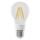One percent of all the lights in the city are LED, the remaining 99% are conventional. Other types are not there. John counted them honestly but he had counted only conventional. After a good dinner he registered numbers and he have notice that from all t
• In the classroomThere are 30 boys and a few girls in the class. In the six months, 28 boys and all girls benefited, which was 95% of all pupils. How many pupils are there in the classroom?
• Acid solutionBy adding 250 grams of a 96% sulfuric acid solution to its 3% solution, we changed its initial concentration to 25%. How many grams of 3% of the acid was used for dilution?
• Diesel fuel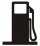In the morning, we refueled and set off. After the first stop, we passed 20% of diesel, after the second 10% of the balance. At the third stop, we had only 9 liters of diesel, and we had to refuel. How big a tank do we have?
• Four numbersThe first number is 50% second, the second number is 40% third, the third number is 20% of the fourth. The sum is 396. What are the numbers?
• Cinema ticketsCinema sold 180 tickets this Thursday, which is 20%. Monday 14%, Tuesday 6%, Wednesday 9%, Friday 24%, Saturday 12%, and Sunday 15%. How many tickets were sold per week?
• Jane classWhen asked how many students are in class, Jane said, if we increase the number of students in our class by a hundred % and then add half the number of students, we get 100. How many students are in Jane's class?
• Sisters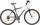Sisters Zuzka Lenka and Vierka has save for a new bike. Zuzka save € 90, Lenka save € 90 and Vierka save € 82. Missing 30% gave them their mother. What is the price of the bike?
• The notebook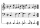After rising by 40%, the notebook cost 10.50 euros. How much did this notebook cost if it increased in price by only 20% instead of 40%?
• Ski class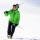Class attend 30 boys and some number of girls. Ski training was attended by 28 boys and all the girls, which was 95% of all students. How many girls attend this class? How many percent is that?
• Fertilizer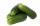Mr. Gherkin use for fertilization 7% solution of fertilizer. 17 liters of it still left. How much water must be added to the solution to make only 3% solution?